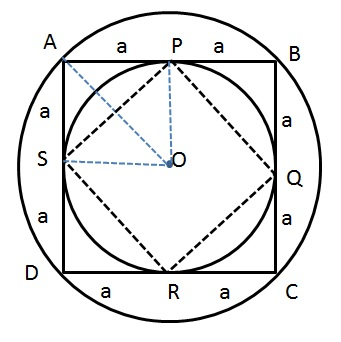# CAT Quantitative Aptitude Questions | Geometry Questions for CAT - Triangles

###### CAT Questions | CAT Geometry Questions | Polygon and Circles

The question is from the topic circles and polygons.It discusses about the ratio of circumference of circle to the polygon that is inscribed in it. CAT Geometry questions are heavily tested in CAT exam. Make sure you master Geometry problems.

Question 35: There are 2 concentric circles, one big and one small. A square ABCD is inscribed inside the big circle while the same square circumscribes the small circle. The square touches the small circle at points P, Q, R and S. Determine the ratio of circumference of big circle to the polygon PQRS.

1. π : 2
2. 2 : π
3. 2 : √2
4. π : √2

## Best CAT Coaching in Chennai

#### CAT Coaching in Chennai - CAT 2021Online Batches Available Now!

##### Method of solving this CAT Question from Circles: Finding out what shape is PQRS and assuming the side of ABCD to be 2a will help to get further answer!Let the side of the square ABCD be 2a.
A line from the centre will bisect the chords AB, BC, CD and DA
Therefore AP = PB = a. Similarly for other sides too.
From the above we can conclude that PQ = QR = RS = SP. Therefore PQRS is also a square.

Radius of big circle = diagonal of the square APOS = a√2
Circumference = 2π * a√2
Now we have to find the side of square PQRS.
We know that radius of small circle = side of the square APOS = a
Consider triangle PSO. PS2 = OP2 + OS2. Therefore, PS = a√2
Circumference of square with side a√2 = 4a√2
Ratio = 2π * a√2 : 4a√2
=> π : 2

The question is "Determine the ratio of circumference of big circle to the polygon PQRS."

##### Hence, the answer is π : 2

Choice A is the correct answer.

###### CAT Coaching in ChennaiCAT 2021Enroll at 49,000/- 44,000/-

Online Classroom Batches Starting Now!

###### Best CAT Coaching in ChennaiPrices slashed by Rs 4000/-

Attend a Demo Class

## CAT Preparation Online | CAT Geometry questions Videos On YouTube

#### Other useful sources for Geometry Question | Geometry Triangles Circles Quadrilaterals Sample Questions

##### Where is 2IIM located?

2IIM Online CAT Coaching
A Fermat Education Initiative,
58/16, Indira Gandhi Street,
Kaveri Rangan Nagar, Saligramam, Chennai 600 093

##### How to reach 2IIM?

Phone: (91) 44 4505 8484
Mobile: (91) 99626 48484
WhatsApp: WhatsApp Now
Email: prep@2iim.com